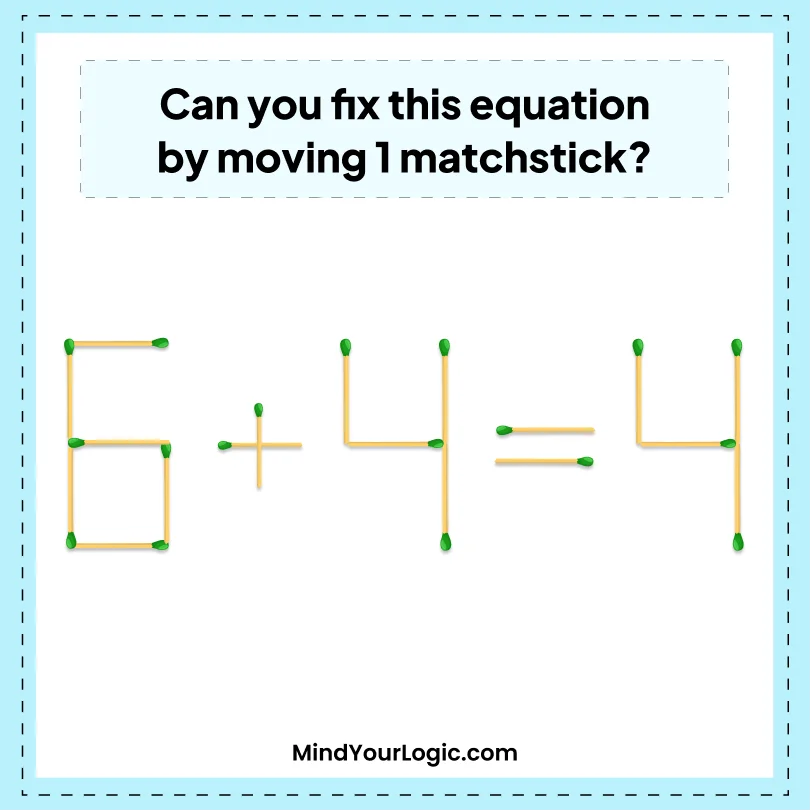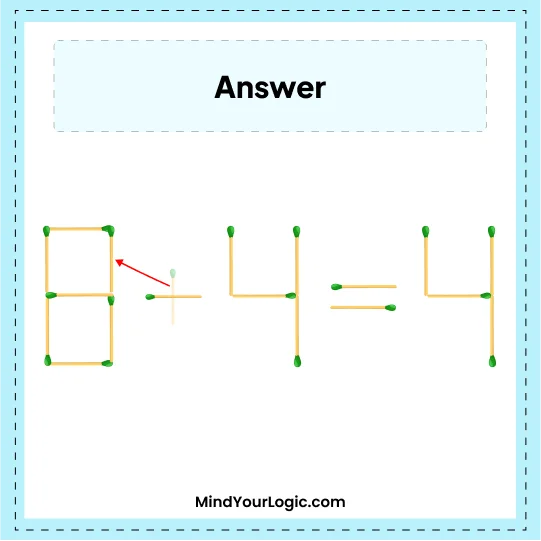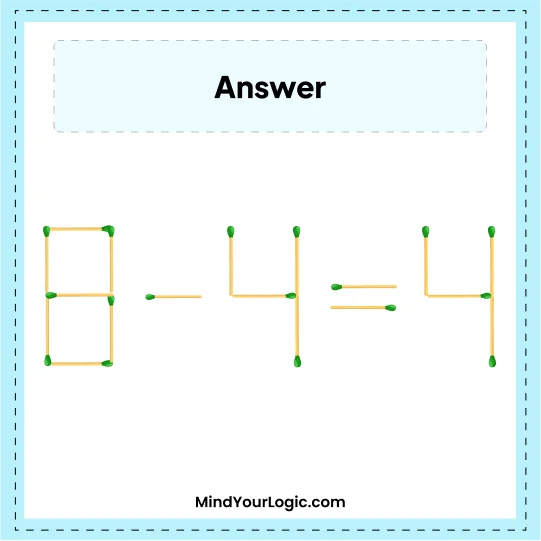# Fix 6 + 4 = 4 By Moving 1 Matchstick Puzzle - Matchstick Puzzle

###### 13.Matchstick Puzzles
`Can you fix this equation by moving 1 matchstick?`•

Explanation :

` Move one matchstick from the + sign to 6, which becomes 8. So, we get the correct equation.`